Processing ......FreeComputerBooks.com Links to Free Computer, Mathematics, Technical Books all over the World

A Computational Introduction to Number Theory and Algebra
Top Free Mathematics Books 🌠 - 100% Free or Open Source!
• Title A Computational Introduction to Number Theory and Algebra
• Author(s) Victor Shoup
• Publisher: Cambridge University Press; 2 edition (2009); eBook (Creative Commons Edition, Version 2.1)
• Hardcover/Paperback 600 pages
• eBook PDF files
• Language: English
• ISBN-10: 0521516447
• ISBN-13: 978-0521516440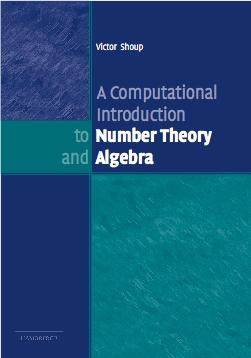Book Description

Number theory and algebra play an increasingly significant role in computing and communications, as evidenced by the striking applications of these subjects to such fields as cryptography and coding theory.

This introductory book emphasizes algorithms and applications, such as cryptography and error correcting codes, and is accessible to a broad audience.

The presentation alternates between theory and applications in order to motivate and illustrate the mathematics. The mathematical coverage includes the basics of number theory, abstract algebra and discrete probability theory.

This edition now includes over 150 new exercises, ranging from the routine to the challenging, that flesh out the material presented in the body of the text, and which further develop the theory and present new applications.

The material has also been reorganized to improve clarity of exposition and presentation. Ideal as a textbook for introductory courses in number theory and algebra, especially those geared towards computer science students.

• Victor Shoup is a computer scientist and mathematician and a Professor in the Department of Computer Science at the Courant Institute of Mathematical Sciences, New York University, focusing on algorithm and cryptography courses.
Reviews, Ratings, and Recommendations: Related Book Categories: Read and Download Links:Similar Books:
•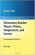Elementary Number Theory: A Computational Approach

This is a book about prime numbers, congruences, secret messages, and elliptic curves that you can read cover to cover. Many numerical examples are given throughout the book using the SageMath mathematical software.

•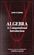Algebra: A Computational Introduction (John Scherk)

This book is a unique approach and presentation, the author demonstrates how software can be used as a problem-solving tool for algebra. It includes many computations, both as examples and as exercises.

•Computational Linear Algebra and N-dimensional Geometry

This undergraduate textbook on Linear Algebra and n-Dimensional Geometry, in a self-teaching style, is invaluable for sophomore level undergraduates in mathematics, engineering, business, and the sciences.

•Applied and Computational Linear Algebra: A First Course

This book is intended as a text for a graduate course that focuses on applications of linear algebra and on the algorithms used to solve the problems that arise in those applications. It uses matrix theory to understand the workings of the algorithms.

•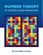Number Theory: In Context and Interactive (Karl-Dieter Crisman)

This is a textbook for an upper-level number theory course, with a clear vision to expose students to the connections to all areas of mathematics, and nearly every concept can be visualized or experimented with using the mathematics software SageMath.

•An Introduction to the Theory of Numbers (Leo Moser)

This book, which presupposes familiarity only with the most elementary concepts of arithmetic (divisibility properties, greatest common divisor, etc.), is an expanded version of a series of lectures for graduate students on elementary number theory.

•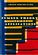Number Theory and Its Applications (Cheon Seoung Ryoo)

Number theory plays a fundamental and important role in mathematics and applied mathematics. This book is based on recent results in all areas related to number theory and its applications.

•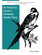An Introductory Course in Elementary Number Theory (Wissam Raji)

This book contains a useful introduction to important topics that need to be addressed in a course in number theory. Proofs of basic theorems are presented in an interesting and comprehensive way that can be read and understood even by non-majors.

•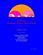Yet Another Introductory Number Theory Textbook (J. A. Poritz)

This introductory number theory textbook has a particular emphasis on connections to cryptology. It broaches the subject of these cryptologic algorithms to develop connections to the topic of number theory.

•Topology of Numbers (Allen Hatcher)

A textbook on elementary number theory from a geometric point of view, as opposed to the usual strictly algebraic approach. A fair amount of the book is devoted to studying Conway's topographs associated to quadratic forms in two variables.

•Magic Squares and Cubes (William Symes Andrews)

This book cover topics such as magic squares, magic cubes, the Franklin squares, magics and Pythagorean numbers, the theory of reversions, magic circles, spheres, and stars, and magic octahedroids, among other things.

Book Categories
 :All CategoriesRecent BooksMiscellaneous BooksComputer LanguagesComputer ScienceData Science/DatabasesElectrical EngineeringJava and Java EE (J2EE)Linux and UnixMathematicsMicrosoft and .NETMobile ComputingNetworking and CommunicationsSoftware EngineeringSpecial TopicsWeb Programming
Other Categories